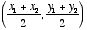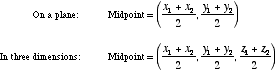# midpoint formula

Midpoint Formulais the formula for the midpoint between points (x1, y1) and (x2, y2). Note that this is simply the average of the x-coordinates and the average of the y-coordinates.